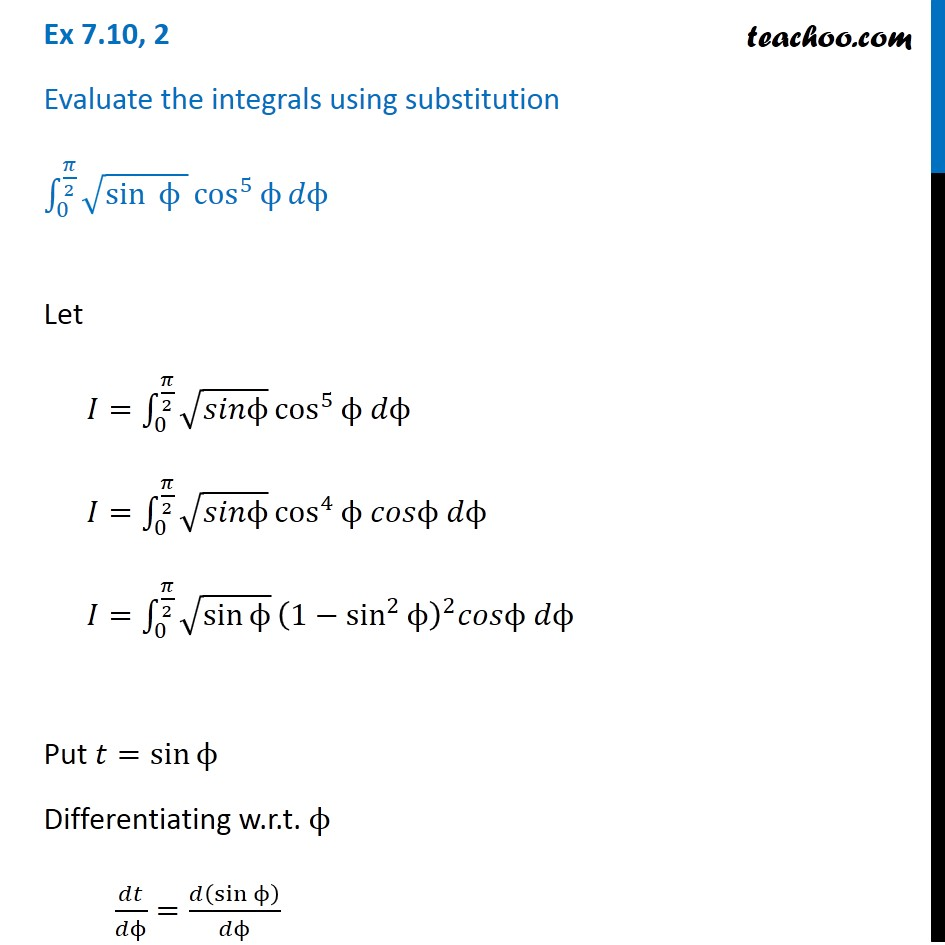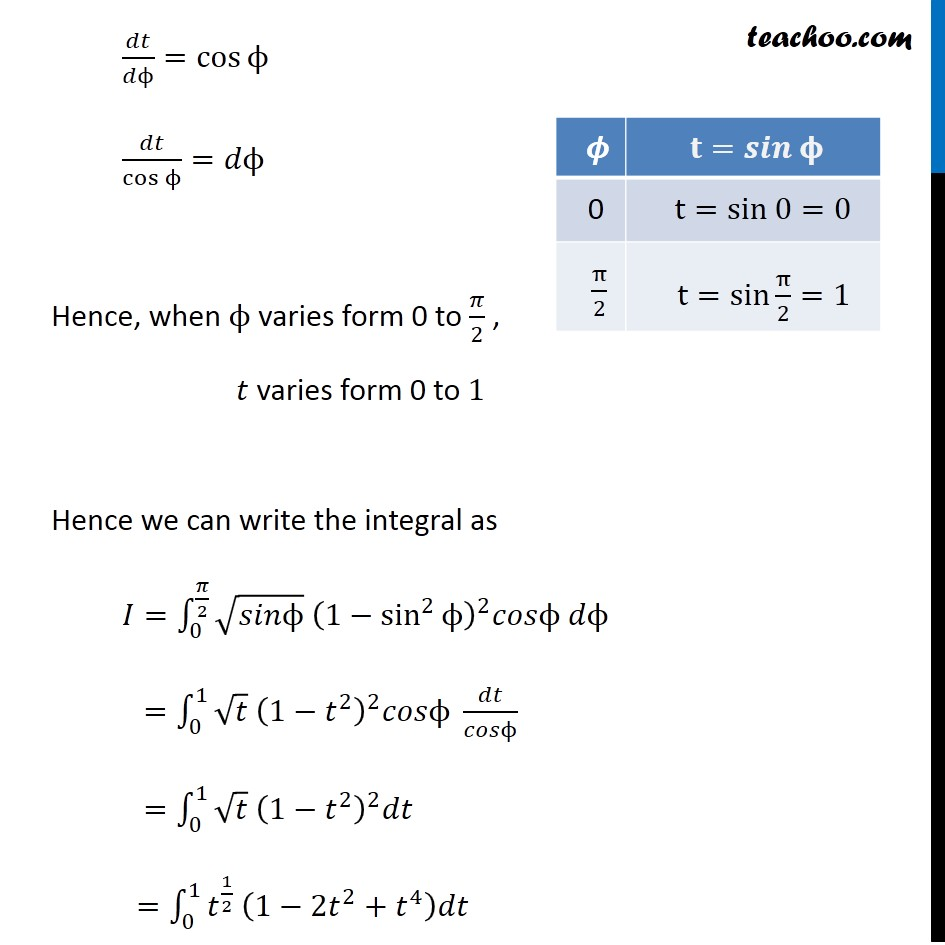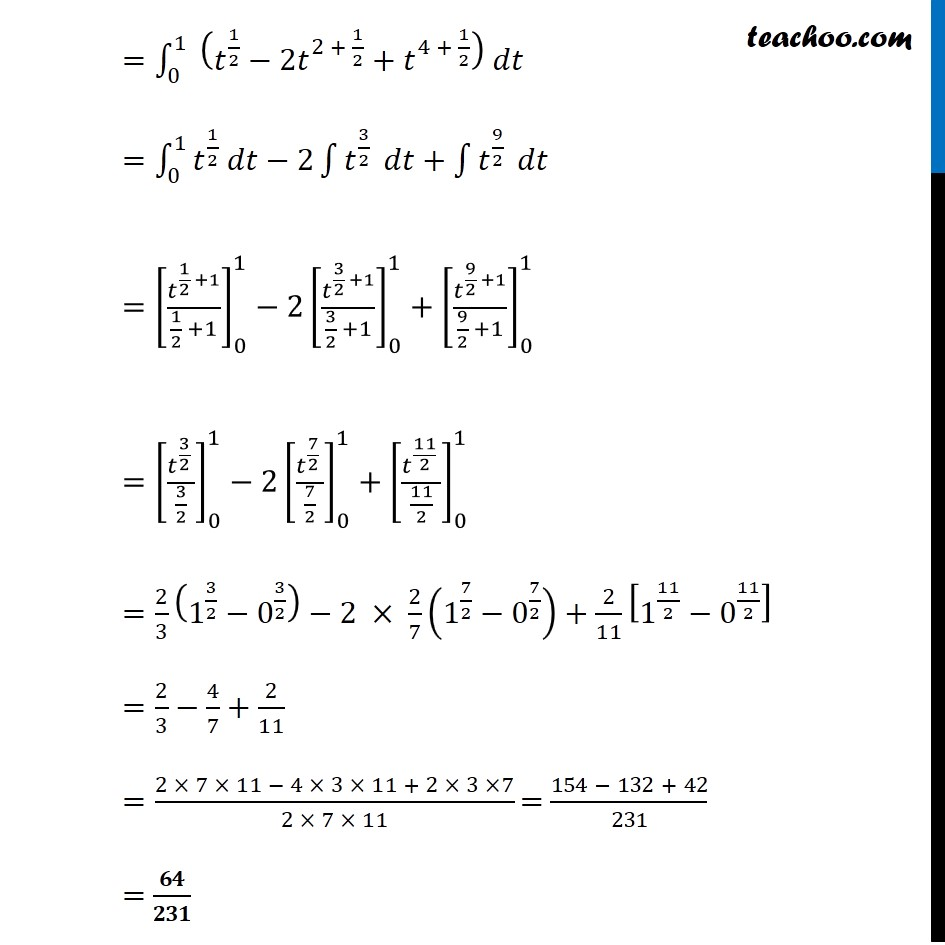Integration Full Chapter Explained - Integration Class 12 - Everything you need1. Chapter 7 Class 12 Integrals
2. Concept wise
3. Definite Integration - By Formulae

Transcript

Ex 7.10, 2 Evaluate the integrals using substitution ∫_0^(𝜋/2)▒〖√(sin⁡〖" " ϕ" " 〗 ) cos^5⁡ϕ 𝑑ϕ〗 Let 𝐼=∫_0^(𝜋/2)▒〖√𝑠𝑖𝑛ϕ cos^5⁡〖ϕ 𝑑ϕ〗 〗 𝐼=∫_0^(𝜋/2)▒〖√𝑠𝑖𝑛ϕ cos^4⁡〖ϕ 𝑐𝑜𝑠ϕ 𝑑ϕ〗 〗 𝐼=∫_0^(𝜋/2)▒〖√(sin⁡ϕ ) (1−sin^2⁡ϕ )^2 𝑐𝑜𝑠ϕ 𝑑ϕ〗 Put 𝑡=sin⁡ϕ Differentiating w.r.t. ϕ 𝑑𝑡/𝑑ϕ=𝑑(sin⁡ϕ )/𝑑ϕ 𝑑𝑡/𝑑ϕ=cos⁡ϕ 𝑑𝑡/cos⁡ϕ =𝑑ϕ Hence, when ϕ varies form 0 to 𝜋/2 , 𝑡 varies form 0 to 1 Hence we can write the integral as 𝐼=∫_0^(𝜋/2)▒〖√𝑠𝑖𝑛ϕ (1−sin^2⁡ϕ )^2 𝑐𝑜𝑠ϕ 𝑑ϕ〗 =∫_0^1▒〖√𝑡 (1−𝑡^2 )^2 𝑐𝑜𝑠ϕ 𝑑𝑡/𝑐𝑜𝑠ϕ〗 =∫_0^1▒〖√𝑡 (1−𝑡^2 )^2 𝑑𝑡〗 =∫_0^1▒〖𝑡^(1/2) (1−〖2𝑡〗^2+𝑡^4 )𝑑𝑡〗 =∫_0^1▒〖 (𝑡^(1/2)−〖2𝑡〗^(2 + 1/2)+𝑡^(4 + 1/2) ) 𝑑𝑡〗 =∫_0^1▒〖𝑡^(1/2) 𝑑𝑡〗−2∫1▒𝑡^(3/2) 𝑑𝑡+∫1▒𝑡^(9/2) 𝑑𝑡 =[𝑡^(1/2 +1)/(1/2 +1)]_0^1−2[𝑡^(3/2 +1)/(3/2 +1)]_0^1+[𝑡^(9/2 +1)/(9/2 +1)]_0^1 =[𝑡^(3/2 )/(3/2)]_0^1−2[𝑡^(7/2 )/(7/2)]_0^1+[𝑡^(11/2 )/(11/2)]_0^1 =2/3 (1^(3/2)−0^(3/2) )−2 × 2/7 (1^(7/2)−0^(7/2) )+2/11 [1^(11/2)−0^(11/2) ] =2/3−4/7+2/11 =(2 × 7 × 11 − 4 × 3 × 11 + 2 × 3 ×7)/(2 × 7 × 11) =(154 − 132 + 42)/231 =𝟔𝟒/𝟐𝟑𝟏

Definite Integration - By Formulae

About the AuthorDavneet Singh
Davneet Singh is a graduate from Indian Institute of Technology, Kanpur. He has been teaching from the past 9 years. He provides courses for Maths and Science at Teachoo.